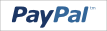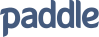Convert tracks into different formats. Also, in this section you can recalculate coordinates from degrees to degrees/minutes (and vice versa) or calculate distances between two points.

Select a file to convert

Select the type of file you want to receive after conversion:

Switch to easy mode.

Coordinate conversion

Enter coordinates (lat, lng) in one of the formats:

 Degrees (example: 41.89014, 12.49244)     Deg.Min (example: 41° 53.409N, 12° 29.546E)     Deg.Min.Sec (example: 41°53'24.5"N, 12°29'32.8"E) Degrees (lat, lng)     Deg.Min (lat, lng)     Deg.Min.Sec (lat, lng)

 Calculate the distance between two points    Enter the coordinates in the following format Degrees (lat, lng):    Point 1   Point 2

 We accept: